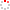NSSC OpenIR  > 空间科学部
 New approach for solving the inverse boundary value problem of Laplace's equation on a circle: Technique renovation of the Grad-Shafranov (GS) reconstruction Li, H. J.; Feng, X. S.; Xiang, J.; Zuo, P. B.; Feng, XS (reprint author), Chinese Acad Sci, Ctr Space Sci & Appl Res, State Key Lab Space Weather, SIGMA Weather Grp, Beijing 100190, Peoples R China. Department 空间科学部 Source Publication JOURNAL OF GEOPHYSICAL RESEARCH-SPACE PHYSICS2013 Volume 118Issue:6Pages:2876-2881 ISSN 2169-9380 Language 英语 Keyword Grad-shafranov Reconstruction Inverse Boundary Value Problems Hilbert Transform Method Of Discrete Vortex Abstract In this paper, the essential technique of Grad-Shafranov (GS) reconstruction is reformulated into an inverse boundary value problems (IBVPs) for Laplace's equation on a circle by introducing a Hilbert transform between the normal and tangent component of the boundary gradients. It is proved that the specified IBVPs have unique solution, given the known Dirichlet and Neumann conditions on certain arc. Even when the arc is reduced to only one point on the circle, it can be inferred logically that the unique solution still exists there on the remaining circle. New solution approach for the specified IBVP is suggested with the help of the introduced Hilbert transform. An iterated Tikhonov regularization scheme is applied to deal with the ill-posed linear operators appearing in the discretization of the new approach. Numerical experiments highlight the efficiency and robustness of the proposed method. According to linearity of the elliptic operator in GS equation, its solution can be divided into two parts. One is solved from a semilinear elliptic equation with an homogeneous Dirichlet boundary condition. The other is solved from the IBVP of Laplace's equation. It is concluded that there exists a unique solution for the so-called elliptic Cauchy problem for the essential technique of GS reconstruction. Indexed By SCI Document Type 期刊论文 Identifier http://ir.nssc.ac.cn/handle/122/4993 Collection 空间科学部 Corresponding Author Feng, XS (reprint author), Chinese Acad Sci, Ctr Space Sci & Appl Res, State Key Lab Space Weather, SIGMA Weather Grp, Beijing 100190, Peoples R China. Recommended CitationGB/T 7714 Li, H. J.,Feng, X. S.,Xiang, J.,et al. New approach for solving the inverse boundary value problem of Laplace's equation on a circle: Technique renovation of the Grad-Shafranov (GS) reconstruction[J]. JOURNAL OF GEOPHYSICAL RESEARCH-SPACE PHYSICS,2013,118(6):2876-2881. APA Li, H. J.,Feng, X. S.,Xiang, J.,Zuo, P. B.,&Feng, XS .(2013).New approach for solving the inverse boundary value problem of Laplace's equation on a circle: Technique renovation of the Grad-Shafranov (GS) reconstruction.JOURNAL OF GEOPHYSICAL RESEARCH-SPACE PHYSICS,118(6),2876-2881. MLA Li, H. J.,et al."New approach for solving the inverse boundary value problem of Laplace's equation on a circle: Technique renovation of the Grad-Shafranov (GS) reconstruction".JOURNAL OF GEOPHYSICAL RESEARCH-SPACE PHYSICS 118.6(2013):2876-2881.